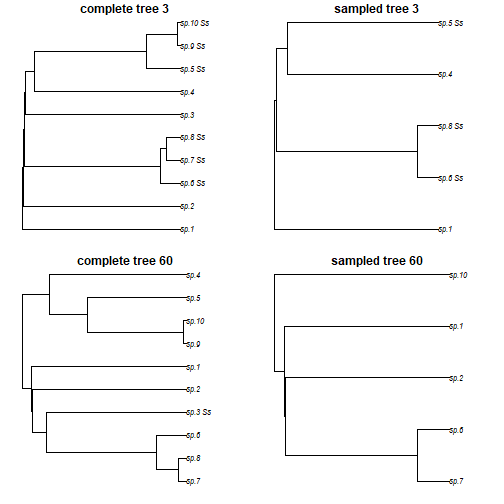Simulation of phylogenetic trees are becoming popular when raising new hypothesis and determining important deterministic forces governing macroevolutionary processes. However, most researches in the area build their own algorithms or use existing simulation tools that are built on different platforms and/or as standalone programs. This makes model selection, replicability, use of cluster computing and pipelining more laborious and prone to failure.

TreeSimGM is an R package available at CRAN under the GPL-2 license for simulating stochastic phylogenetic trees making use of a general and flexible macroevolutionary model. More specifically, with TreeSimGM you can:

• specify any desired probability distribution for the *waiting times until speciation and extinction * (e.g. age-dependent speciation/extinction).

• allow for lineage specific changes in the waiting time to speciation and/or extinction. Meaning that the waiting times to speciation / extinction may be scaled in different parts of the tree allowing for clade-dependent diversification processes on top of the chosen speciation and extinction processes.

• define the speciation mode, i.e. if one descendant species inherits the age of the ancestor species (we call it the asymetric speciation mode), or if both descendant species are new species of age 0 (we call it the symmetric speciation mode).

• use different stopping assumptions, i.e. if the simulated trees have a specific number of extant tips `sim.taxa()`, or if a user-specified stem age is simulated `sim.age()`.

• have complete and incomplete sampling that can be uniform or proportional to pendant branch lengths.

Such generality not only allows you to simulate stochastic phylogenetic trees assuming several popular existing models, such as the Yule model, the constant rate birth-death model, and proportional to distinguishable arrangement models, but also allows you to formulate and explore new macroevolutionary models.

This document introduces you to TreeSimGM's and elucidates with examples how to simulate trees under different models and settings. If you would like to reproduce the exact same result, please use `set.seed(1987)`, since most of the trees presented here are generated under stochastic processes.

## Stopping Assumptions

There are only two main functions in TreeSimGM: `sim.age()` and `sim.taxa()` which are used to simulate trees that contain a certain age or number of living tips respectively. To simulate trees with an age of 2 under a Yule model (exponentially distributed waiting time until speciation with rate 1.2) is simple. After having loaded `library(TreeSimGM)`, generate one single tree and plot it, using `sim.age()`.

``````tree <- sim.age(age=3,numbsim=1,"rexp(1.2)")
plot(tree[], main="Simulated tree of age 3")
``````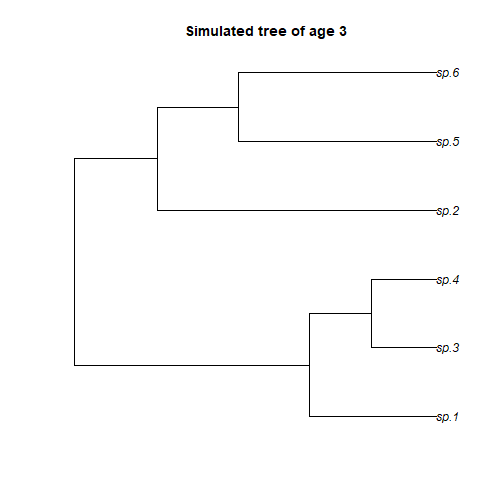Similarly, a single tree simulated until reaching 5 living tips, under the same model as before, is generated by the function `sim.taxa()`.

``````tree <- sim.taxa(numbsim=1, n=5, waitsp="rexp(1.2)")
plot(tree[], main="Simulated tree with 5 living tips")
``````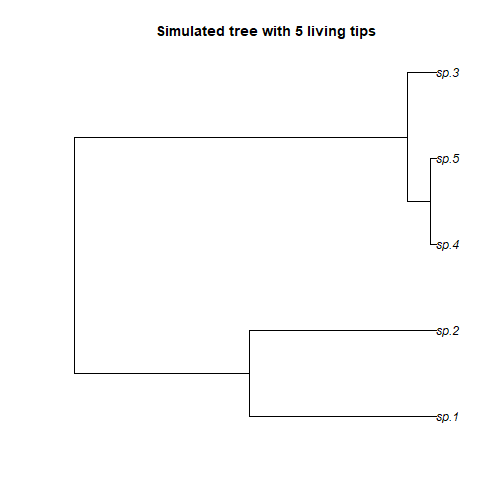However, when simulating trees with `sim.taxa()` one needs to be aware of potential biases promoted by the stopping assumptions. It could be that, by chance, a specific number of living tips, let's say `n=5`, coexisted more than once through the time of tree evolution. If you sample trees at every first time it achieves 5 living tips, you might be biasing the simulation towards young trees. It could be that a tree reaches 5 tips but grows and at another timepoint exhibits 5 tips again. In this case, all trees with 5 tips should have a positive probability of existing, and sampling only the first one would then generate the small trees bias. This problem could be solved by simulating trees that go until m coexisting tips ( with m>>n such that chances are very small that a tree on m tips shrinks again to a tree on n tips) and thereafter sample uniformly among all time points that this tree reached 5 tips. This can be done by setting `m=10` (m is the tip number in the final tree) and gsa=TRUE. Keep in mind that this only is necessary if there is an extinction process taking place.

``````tree <- sim.taxa(numbsim=1, n=5, m=10, waitsp="rexp(1.2)", waitext="rexp(1.1)", gsa=TRUE)
plot(tree[], main="Returned tree with 5 living tips from simulated tree with 10 tips")
``````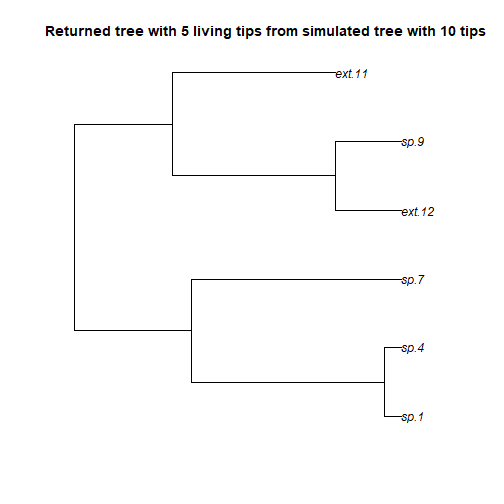When simulating with `sim.age` not always are trees returned. More specifically, if the tree is extinct before the defined age, the output is a ZERO. A ONE is returned if only one extant species exists. This can happen if speciation is too weak.

Simulating 100 trees under `sim.age()` with exponential speciation and extinction will not always return phylogenetic trees.

``````tree <- sim.age(age=2, numbsim=100, waitsp="rexp(1)", waitext="rexp(1)")
table(unlist(lapply(tree, class)))
#>
#> numeric   phylo
#>      66      34
``````

To access the last simulated tree.

``````tree[]
#>  0
``````

It happens to be a tree that went extinct before reaching the defined age.

## Defining Speciation and Extinction Distributions

In order to simulate different macroevolutionary models, `TreeSimGM` allows you to define different distributions for the waiting times until speciation (parameter `waitsp`) and extinction (parameter `waitext`). This can be done in two ways, by using a string with the chosen waiting time distribution and parameters or by using a function that generates a single waiting time per call.

#### Setting waiting times with strings

As shown in the previous examples, we can determine the waiting times by parsing to the respective parameter (either `waitsp` or `waitext`) the name of the random number generator function under the desired probability function and its parameters except for the first. As we need only one randomly generated waiting time per draw, this first parameter of the random number generator function (always the number of observations) is to be omitted from the input string. For example, let's imagine we would like to use an exponential waiting time until speciation. To know its parameters, we can run `?rexp`.

You see that `rexp` takes basically two arguments `n` (number of observation) and `rate` (what we need to specify). Thus to simulate a tree with exponential waiting times with speciation rate of 0.9 the waitsp argument should be `waitsp=rexp(0.9)`. To simulate a tree with weibull waiting time until speciation and exponential waiting time until extinction, first let’s look at the random generation function for the weibull distribution.

``````?rweibull
``````

From the R Documentation we know that `rweibull(n, shape, scale = 1)`, thus on `waitsp` we should only inform `rweibull(shape, scale)`. Let’s take `shape=0.4` and `scale=3` and the extinction process as `rexp(0.1)`.

``````set.seed(1989)
tree <- sim.age(3,1,"rweibull(0.4,3)", "rexp(0.1)")
plot(tree[])
``````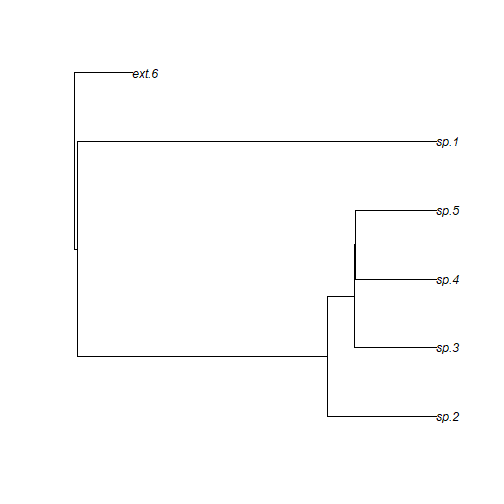For this input method, any probability density function available in R can be used.

#### Setting waiting times with user defined function

To gain flexibility, we can simulate trees using own functions instead of strings as waiting times input. Let’s for example simulate a tree under the same conditions as the previous example. Note that here, we need to add all parameters necessary for the distribution function.

``````set.seed(1989) # setting the same seed as before...
tree_funk <- sim.age(3,1,waitsp=function()rweibull(1,0.4,3), waitext=function()rexp(1,0.1))
``````

This should generate an identical tree, and indeed they are.

``````identical(tree, tree_funk)
#>  TRUE
par(mfrow=c(1,2))
plot(tree[], main="tree (strings)")
plot(tree_funk[], main="tree_funk (function)")
``````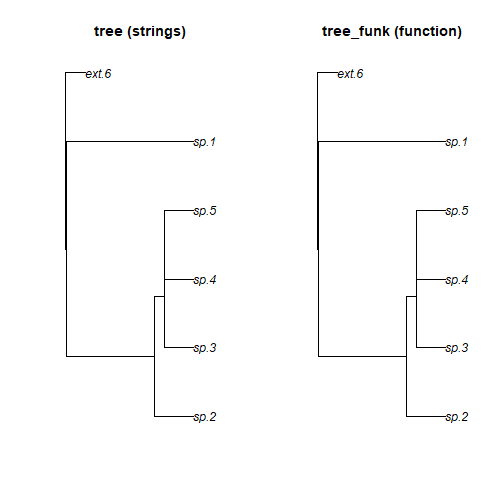Now let's explore the generality and advantage of this model and produce a tree based on our own defined waiting time rules! In our waiting time function, we choose to have exponentially distributed waiting times until speciation that are limit to be at least 0.5! If they are smaller than 0.5, the waiting time will be 0.5. Remember that this function needs to generate one single number.

Here is our waiting time until speciation function.

``````waitspfunk <- function() {
wt=rexp(1,1.5)
if(wt<0.5){wt=0.5}
return(wt)
}
``````

Now we plug `waitspfunk` in our function (you can also define it directly).

``````funk_tree <- sim.age(age=2, numbsim=1, waitsp=waitspfunk)
plot(funk_tree[])
``````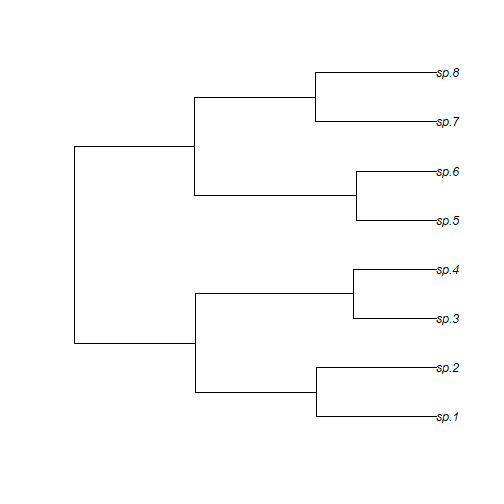`waitext` will work the same way. You can also use functions to define shiftstregths, but we will get into that later…

## The Speciation Modes

On top of the chosen distribution for speciation and extinction, the speciation mode can be chosen. A speciation event is defined as when one species splits into two. Two different modes at these splits are possible: (i) symmetric, where for every speciation event new waiting times until speciation and extinction are drawn for both daughter lineages; and (ii) asymmetric, where a speciation event results in one species with new waiting times, and another that carries the extinction time and age of its ancestor. The symmetric mode can be seen as an vicariant or allopatric process where divided populations undergo equal evolutionary forces while the asymmetric mode could be seen as a peripatric speciation where a mother lineage continues to exist.

Symmetric trees are simulated by default `symmetric=TRUE`. To simulate asymmetric trees set `symmetric=FALSE`.

To stress these speciation modes, let’s use a special model, namely one where all species speciate after 2Myr and go extinct after 2.5Myr. For this we can use `sim.age(age=10)` and the way we input functions as we just learned.

``````symmetric_tree <- sim.age(age=10, numbsim=1, waitsp=function()2, waitext=function()2.5, symmetric=TRUE)
asymmetric_tree <- sim.age(age=10, numbsim=1, waitsp=function()2, waitext=function()2.5, symmetric=FALSE)
par(mfrow=c(1,2))
plot(symmetric_tree[], main="Symmetric tree")
plot(asymmetric_tree[], main="Asymmetric tree")
``````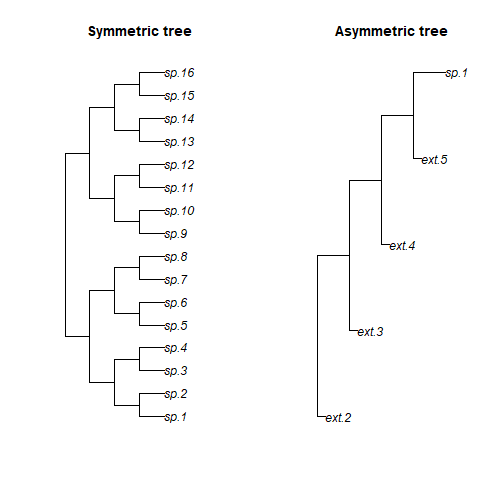This makes clear how these two mechanisms work. While on the symmetric mode, after every speciation event (2Myr) two new species are generated giving thus no chance for a deterministic extinction (2.5Myr) to happen, on the asymmetric mode how the “mother” lineage goes extinct after 0.5Myr after the speciation event that generate a new lineage that survives one next timestep.

## Lineage-Specific Changes

Lineage-specific changes are changes in the waiting times (speciation or extinction) that are hereditary, i.e. passed on to descending new species. To specify a shift (i.e. change) on speciation or extinction the arguments `shiftsp` and `shiftext` are used respectively. Each is a list containing `prob` and `strength` (hereafter `shiftXX\$prob` and `shiftXX\$strength` for a generalization of the speciation or extinction process).

In a simulated tree, the probability of a shift happening when a new species is formed is determined by `shiftXX\$prob`, which by default is ZERO (meaning no shift) and should range within interval[0,1]. In case a shift happened, the strength of this shift will be determined by `shiftXX\$strength`, that works similarly to `waitsp`, i.e. as a string or a function. The value obtained from `shiftXX\$strength`, will be then multiplied by the speciation and/or extinction time (depending if we are using `shiftsp` and/or `shiftext`). This scaling factor will perpetuate to all descending species from this lineage, until a new shift happens that overwrites the inherited shift.

Note that a shift strength > 1 will increase the waiting time while a shift strength < 1 will decrease the waiting time.

Let us simulate Yule trees (speciation rate of 1.2) with shifts happening with a probability of 10% and a scaling factor f chosen uniformly between [0.3, 0.5], meaning that shifted species will have a higher speciation rate.

``````tree <- sim.age(age=2, numbsim=1, waitsp="rexp(1.5)", shiftsp=list(prob=0.1, strength="runif(0.3,0.5)"))
plot(tree[], main="Yule tree with speciation shifts")
``````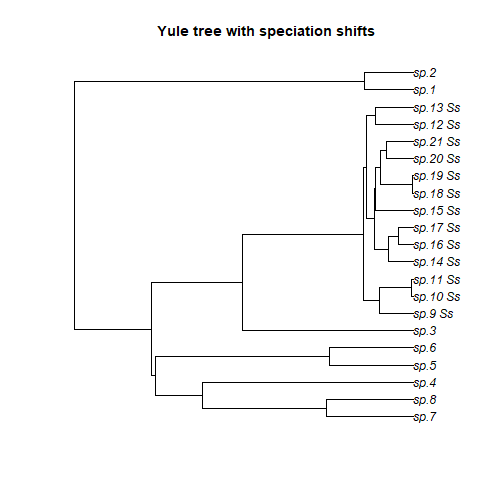One can spot how 'speciation shifted species', i.e. labeled with “Ss” have a much shorter waiting times until speciation.

By default `tiplabel=c("sp.", "ext.","Ss", "Se")`. It is a vector of 4 strings/labels that will be given for each tip that  survived until the present;  got extinct  experienced speciation shifts or  experienced extinction shifts along the way to the root. An automatic sequential number is added to the chosen label.These labels can be changed using the argument `tiplabel`.

Let's now simulate 5 trees under the constant birth-death models (i.e. exponentially distributed waiting times until speciation and extinction) but adding changes in the waiting time to speciation and extinction. This time, we will change these labels.

For this, we will alter a bit the stopping assumption and use `sim.taxa()` for a change.

``````tree <- sim.taxa(numbsim=5, n=6, waitsp="rexp(1)", waitext="rexp(0.5)",
shiftsp=list(prob=0.1, strength=function()0.5),
shiftext=list(prob=0.1, strength=function()0.1 ),
tiplabel=c("living bird ", "ext bird ","SPshift", "EXTshift"))
plot(tree[], main="crBD tree with speciation and extinction shifts")
``````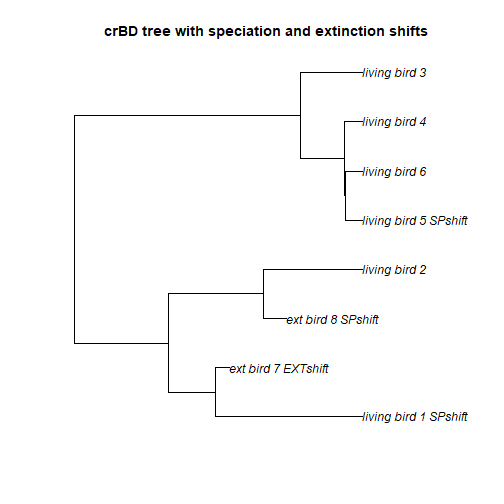Note that here, we used deterministic shifts, meaning that every time a speciation shift happens the waiting times until speciation were scaled by 0.5 and every time a extinction shift happens the waiting times until extinction were scaled by 0.1, which results in a very low value, thus probably all species that suffered extinction shift will go extinct sooner or later.

## Tracking shifts

From the example simulated tree above, let's look into details regarding the shifts.

To know how many trees we simulated

``````length(tree)
#>  5
``````

Let us explore the first one (same as plotted above) regarding rate shifts. Instead of counting the labels containing “shifts”, we now use another method.

``````t1 <- tree[]
t1\$shifted.sp.living
#>      LivingSpecies shift
#> [1,]             1     1
#> [2,]             2     0
#> [3,]             3     0
#> [4,]             4     0
#> [5,]             5     1
#> [6,]             6     0
``````

This lists all living species, and the ones containing a shift=1 are the living species with ancestors having underwent a shift on the speciation process. The living species that contain a shift on the speciation are:

``````t1\$shifted.sp.living[t1\$shifted.sp.living[,2]==1,1]
#>  1 5
``````

Similarly, one can quantify the shifts on extinction on the living species with `\$shifted.ext.living` or the shifts on the extinct species with `\$shifted.sp.extinct` and `\$shifted.ext.extinct`.

As an example, lets account for which and how many living species suffered shifts (no matter if on the speciation or extinction process)

``````#which?
shifted_living <- unique(t1\$shifted.sp.living[t1\$shifted.sp.living[,2]==1,1], t1\$shifted.ext.living[t1\$shifted.ext.living[,2]==1,1])
print(shifted_living)
#>  1 5
#howmany?
length(shifted_living)
#>  2
``````

Now let’s look deeper into these shifts. The scaling factors applied to every node are stored at `\$shiftsp` and `\$shiftext` for speciation and extinction shifts respectively. If we have had not only one possible shift strength, these could look more interesting, but for the sake of simplicity, let's stay with the case study we are looking at and look at its shifts on speciation

``````t1\$shiftsp
#>       node strength
#>  [1,]    9      1.0
#>  [2,]   10      1.0
#>  [3,]   11      1.0
#>  [4,]   12      1.0
#>  [5,]   13      1.0
#>  [6,]   14      1.0
#>  [7,]    1      0.5
#>  [8,]    7      1.0
#>  [9,]    8      0.5
#> [10,]    2      1.0
#> [11,]   15      1.0
#> [12,]    3      1.0
#> [13,]   16      1.0
#> [14,]    4      1.0
#> [15,]    5      0.5
#> [16,]    6      1.0
``````

Since all branches have the same shift, it is difficult to know if all the 3 living species that present a speciation shift inherited it from an common ancestor or if they had a new shift occurring every time.

To look at this, we can use the function `track.shift()` where the first argument defines if we want to track a speciation “sp” or an extinction “ext” shift. Having defined that, we just need to inform the tree object and the node we would like to track.

``````track.shift(shift="sp", tree=t1, node=4)
#>  "sp"
#> 13 14  4
#>  1  1  1
track.shift(shift="sp", tree=t1, node=5)
#>  "sp"
#>  13  14  15   5
#> 1.0 1.0 1.0 0.5
track.shift(shift="sp", tree=t1, node=6)
#>  "sp"
#> 13 14 15  6
#>  1  1  1  1
``````

The result shows us all shifts in speciation between the root and a specific node or tip in the simulated tree, named by its node numbers. Our quick analysis tells us that living bird 5 and 6 share a “shifted ancestor” while living bird 3 does not.

## Incomplete Sampling

To generate trees that are incompletely sampled, use the argument `sampling`. `sampling` is a list containing the sampling fraction `sampling\$frac` and a boolean `sampling\$branchprop` defining if the sampling should be proportional to the pendant branch lengths. Default is `sampling\$branchprop=FALSE`, in the case `sampling\$branchprop=TRUE`, longer branches would be more likely to be sampled. If `sampling\$frac` is smaller than 1, one sampled tree is returned, and the full tree is further returned inside the tree object as `\$beforesampling`. For the sampled tree, shift information can only be visualized through the tip labels, a complete shift history can be retrieved at the full tree \$beforesampling. sampling=list(frac=1, branchprop=FALSE) is default.

Let's assume we would like to get 100 trees with 5 living tips, while assuming that we can sample only 50% for all extant tips. To do this, we will use an asymmetric age-dependent (weibull distribution) speciation process for the speciation without any extinction process and use a speciation shift probability of 30% with strength that ranges uniformly between 0.5 and 0.9 (meaning that we have a shortening of waiting times until speciation for shifted species).

``````tree <- sim.taxa(100, 5, waitsp="rweibull(0.5,2)", symmetric=FALSE, shiftsp=list(prob=0.3, strength="runif(0.5,0.9)"), sampling=list(frac=0.5, branchprop=FALSE))
``````

From these 100 simulated trees, lets plot two of them, with the complete tree next to the sampled tree

``````par(mfrow=c(2,2), mai=c(0.2,0.2,0.2,0.2))
plot(tree[]\$beforesampling, main="complete tree 3")
plot(tree[], main="sampled tree 3")
plot(tree[]\$beforesampling, main="complete tree 60")
plot(tree[], main="sampled tree 60")
``````